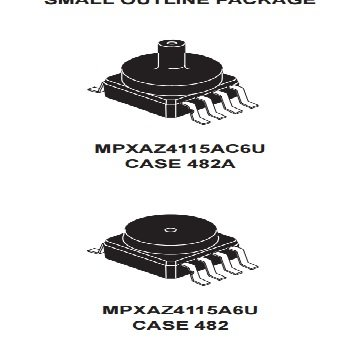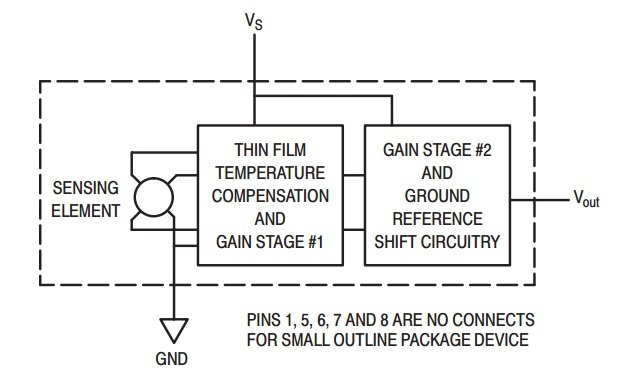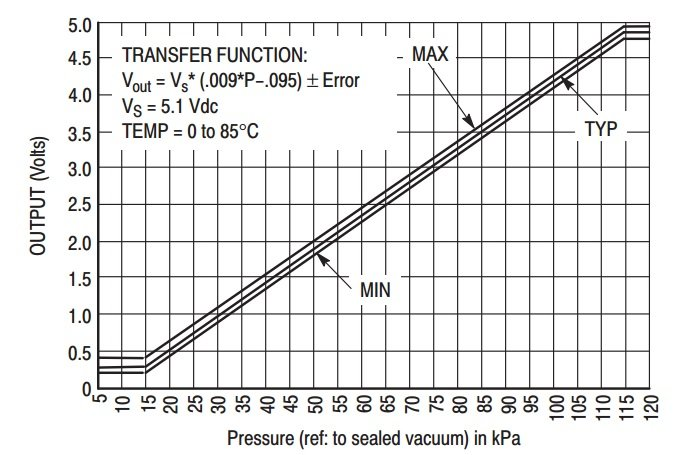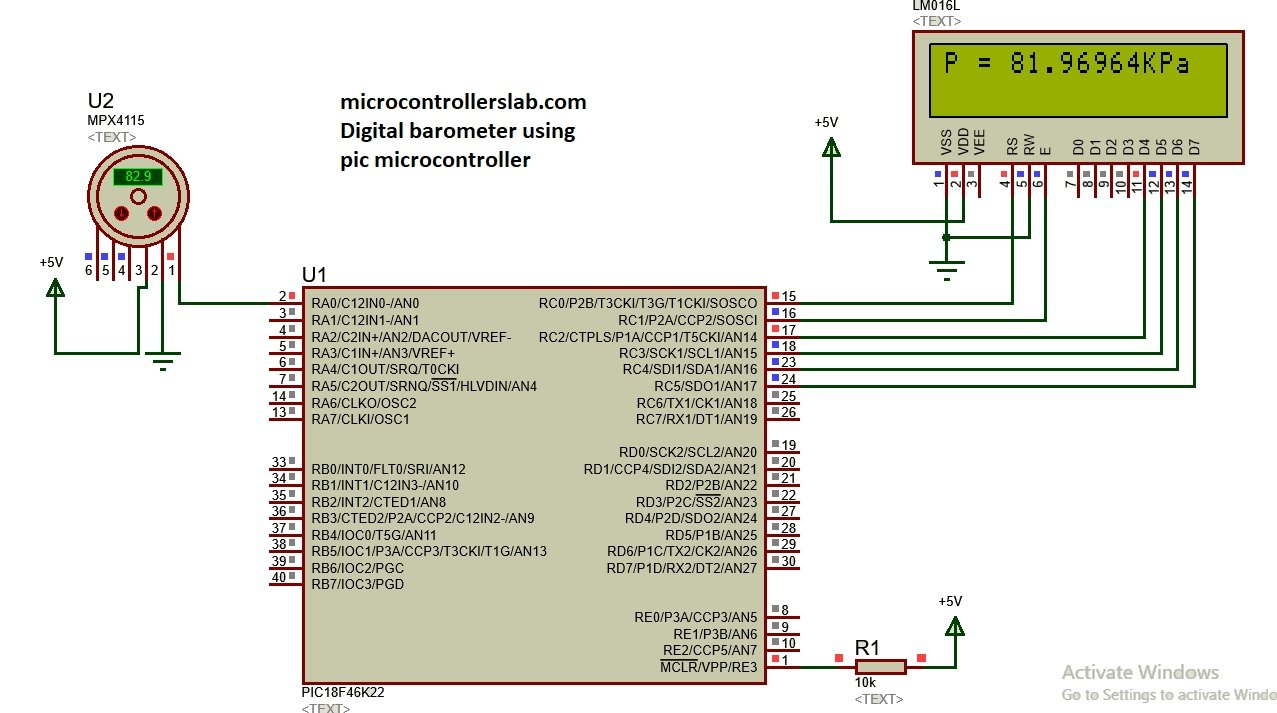# MPX4115A pressure sensor interfacing with pic microcontroller – Digital Barometer

Digital Barometer using pic microcontroller: In this tutorial, you will learn how to interface MPX4115A pressure sensor with pic18f46k22 microcontroller? How to use MPX4115A pressure sensor to design digital Barometer? What are the features of MPX4115A pressure sensor? How MPX4115A pressure sensor works? How to measure output voltage of MPX4115A pressure sensor with the help of pic18f46k22 pic microcontroller and convert this measured voltage into pressure and display measured pressure value on liquid crystal display? Now lets start with basic introduction and working of MPX4115A pressure sensor which is necessary to understand. Before interfacing this pressure sensor with pic microcontroller, we should know how this sensor works and what is the output of this sensor?  you may also like to check these sensors interfacing tutorials with pic microcontroller:

### Introduction to MPX4115A pressure sensorMPX4115A pressure sensor is a integrated on chip pressure sensor. It consists of piezoresistive transducer which converts pressure into dc voltage.  It can measure pressure from 15 kilo pascal to 115 kilo pascals. And output of sensor ranges from 0.2 volt to 4.8 volt according to pressure applied to sensor.  Followings are the main feature of this sensor:

Followings are the main applications of this sensor:

• Automotive industry
• engine control
• weather stations
• And many others

The internal block diagram of MPX4115A pressure sensor is shown below. It has three pins +5 volt input pin, ground pin and output pin and we measure the output voltage from this pin and convert it back in pressure using the formula which is given in data sheet. We provide pressure to a sensing element.  To design a microcontroller based barometer it is a very useful pressure sensor.Graph below shows the relationship between output voltage and input pressure. It can easily observed that both has somehow linear relationship with each other and formula for voltage to pressure conversion is also given in the graph.So according to above graph the minimum pressure we can measure with this sensor is 15kPa and maximum is 115Kpa.  Because below, 15KPa output voltage of sensor is zero and on above 115KPa output voltage remains constant.  So we can easily easily design digital barometer with the help of this pressure sensor. Because we already know that how to measure voltage with the help of pic18f46k22 microcontroller and by using above conversion formula we can easily convert voltage into pressure.  Formula below shows the relationship between output voltage of sensor and input pressure.

Vout = Vs x ((0.009 x P) – 0.095) ± Error

### MPX4115A pressure sensor interfacing with pic microcontroller

In this project ,we are designing digital barometer using pic18f46k22 microcontroller. So now lets see how to connect MPX4115A pressure sensor with pic microcontroller.  To interface this sensor with pic microcontroller, we just need to connect output pin of MPX4115A pressure sensor with analog input pin of pic microcontroller as shown below. I have connected analog pin zero of pic18f46k22 with output pin of barometer sensor. I have also connected lcd with pic18f46k22 microcontroller to display measured value of pressure. If you don’t know how to interface LCD with pic microcontroller. Check this lcd interfacing with pic16f877a microcontroller articleSo above simulation shows the measured value of pressure on LCD.

### Code for pressure sensor interfacing with pic microcontroller

Code for pressure sensor interfacing with pic microcontroller is given below. Code is written using Mikro c for pic.

```// LCD module connections
sbit LCD_RS at LATC0_bit;
sbit LCD_EN at LATC1_bit;
sbit LCD_D4 at LATC2_bit;
sbit LCD_D5 at LATC3_bit;
sbit LCD_D6 at LATC4_bit;
sbit LCD_D7 at LATC5_bit;

sbit LCD_RS_Direction at TRISC0_bit;
sbit LCD_EN_Direction at TRISC1_bit;
sbit LCD_D4_Direction at TRISC2_bit;
sbit LCD_D5_Direction at TRISC3_bit;
sbit LCD_D6_Direction at TRISC4_bit;
sbit LCD_D7_Direction at TRISC5_bit;
// End LCD module connections

float pressure;
unsigned char txt;

void main() {
OSCCON=0x66; //Configure to use 8MHz internal oscillator.
ANSELC=0X00;
ANSELA=0XFF;
TRISA.RA0 = 1; // Configure RA0 pin as input

Lcd_Init(); // Initialize LCD
Lcd_Cmd(_LCD_CLEAR); // Clear display
Lcd_Cmd(_LCD_CURSOR_OFF); // Cursor off

Lcd_Out(1, 1, "microcontrollers"); // Display "StudentCompanion"
Lcd_Out(2, 1, "lab.com"); // Display "Thermometer"
Delay_ms(2000); // 2 Second delay
Lcd_Cmd(_LCD_CLEAR); // Clear display

do {
pressure = (pressure + 0.475)/0.0475;
FloatToStr(pressure, txt);
Lcd_Out(1, 1, "P = ");
Lcd_Out_Cp(txt);
Lcd_Out_Cp("KPa");

} while(1);

}```

### 10 thoughts on “MPX4115A pressure sensor interfacing with pic microcontroller – Digital Barometer”

1. Can i use this sensor for measuring pressure of liquid (e.g: Pressure of water)
I mean could this sensor be in water
thanks……………….

• Thank you for your response
So this sensor can only measure the atmosphere pressure ??
if you may: what other sensors can be used to measure water level except ultrasonic

2. Sir, can we interface weight sensor & color sensor with pic18f4550?

3. Having read this I thought it was really enlightening.
I appreciate you spending some time and energy to put this content together.
I once again find myself spending a significant amount of time both
reading and commenting. But so what, it was still worth it!

4. that is nice but i hasn’t the micro c compiler
i want the code for MALAB IDE….